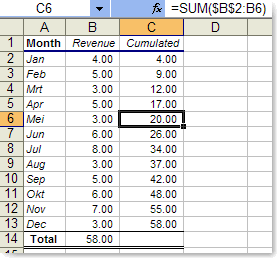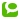You all know that +, -, * and / are operators, and you use them extensively in Excel. There is one operator you also use regularly, but you might not be aware that it's also an operator. I'm talking about the : (colon).
A colon 'joins' 2 references; e.g. SUM(A2:A13) will summarize all cells starting from A2 up to and including A13. You knew that.
When you start playing with the references before and after the colon, the fun begins.
Let's say you need to have a running total. Here's a screenshot.Notice the formula for the running total in C6: SUM(\$B\$2:B6). This formula is exactly the same (relatively speaking) in all cells from C2 up to C13.
\$B\$2 is always the same fixed cell, B6 'varies' according to the cell.

I told you before that the INDEX function (as well as the OFFSET function) results in a reference. This means you can use them in combination with the colon-operator too.
When working with Names, they become even more useful.
Suppose you define a name for the 12 month revenue range,
and call B2:B13 'Revenue'.
Now you can e.g. calculate a quarterly total using INDEX:The formula for the Q-total is easy (it looks complex, but only because I calculate the beginning and ending month based on the quarter):

• =SUM(INDEX(Revenue,(Quarter-1)*3+1):INDEX(Revenue,(Quarter-1)*3+3))
Next time you use the colon in Excel, think about other uses for it.

Category:  Microsoft Excel  |Technorati: ,